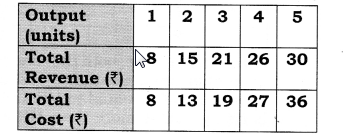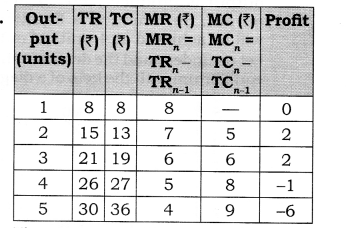# NCERT Solutions Class 12 Economics Chapter-9 (Producer Equilibrium)

NCERT Solutions Class 12 Economics from class 12th Students will get the answers of Chapter-9 (Producer Equilibrium) This chapter will help you to learn the basics and you should expect at least one question in your exam from this chapter.
We have given the answers of all the questions of NCERT Board Economics Textbook in very easy language, which will be very easy for the students to understand and remember so that you can pass with good marks in your examination.

Class 12 Economics

Chapter-9 (Producer Equilibrium)

Questions and answers given in practice

Chapter-9 (Producer Equilibrium)

Question 1. What conditions must hold if a profit- maximizing firm produces positive output in a competitive market?
Or [6 Marks]
Explain the producer’s equilibrium with MR/MC approach (when Price remains constant with the rise in output).
Or
Explain the conditions of a producer’s equilibrium in terms of marginal cost and marginal revenue. Use diagram
[CBSE Sample Paper 2012, 13] Or
Why is the equality between marginal cost and marginal revenue necessary for a firm to be in equilibrium? Is it sufficient to ensure equilibrium? Explain. [CBSE 2015}
Or
Elaborate the implication of the conditions of equilibrium of a firm.
[CBSE Sample Paper 2016]

Answer: The conditions must hold if a profit maximizing firm produces positive output in a competitive market when price is constant’under MR/MC approach is determined where,
(i) MR = MC (ii) MC must be rising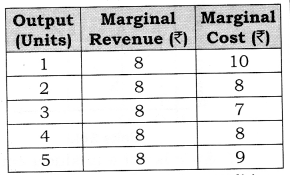According to Table, both the conditions of equilibrium are satisfied at 4 units of output. MC is equal to MR and MC is rising. MC is more than MR when output is produced after 4 units of output. So, Producer’s Equilibrium will be achieved at 4 units of output. However, MR is equal to MC at 2 units of output also. But, second condition is not fulfilled here.
Let us understand the determination of equilibrium with the help of a diagram: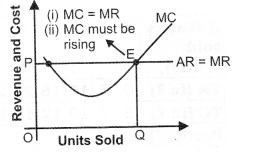Producer’s Equilibrium is determined at OQ level of output corresponding to point E as at this point, MC = MR and MC curve cuts MR curve from below. In Figure, output is shown on the horizontal axis and revenue and costs on the vertical axis. Producer’s equilibrium will be determined at OQ level of output corresponding to point E because at this, the following two conditions are met:
(i) MC = MR;
(ii) MC curve cuts the MR curve from below.
When MR > MC, then producer will continue to produce as long as MR becomes equal to MC. It is so because firm will find it profitable to raise the output level.
When MR < MC, then producer will cut down the production as long as MR becomes equal to MC. It is so because firm will find it unprofitable to produce an extra unit. So, it starts reducing the level of output till MR = MC.

Question 2. Can there be a positive level of output that a profit-maximizing firm produces in a competitive market at which market price is not equal to marginal cost? Give an explanation. [NCERT on Page 66][6 Marks] Or
Explain why will a producer not be in equilibrium if the conditions of equilibrium are not met. [AI 2015]

Answer: The profit maximizing level of output is always determined where,
(i) MR = MC
(ii) MC must be rising. In other words, where price is equal to MC.
If price is not equal to MC, profit maximizing condition cannot hold. It can be explained with the help of the following two cases:
Case 1: Price Greater Than MC
(i) In the given figure at output level q2, the market price is greater than marginal cost.
(ii) To show that q2 is not a profit maximizing level of output, we have taken q3 output level, which is right of qr
(iii) Suppose the firm increases its output level from q2 to qr The increase in total revenue of the firm from this output is the market price multiplied by the change in quantity (ATR = market price x AQ), that is, the area of rectangle q2q3CB.
(iv) On the other hand, the increase in total cost with this, increase in output is the area of the region q2q3XW.
(v) But, a comparison of the two area shows that the firm’s profit is higher when output level is q3 rather than q1 So, q2 is not a profit maximizing level of output.
Case 2: Price Less Than MC
(i) In the given figure at output level q2, the market price is less than marginal cost.
(ii) To show that q2 is not a profit maximizing level of output, we have taken q3 output level, which is left of qr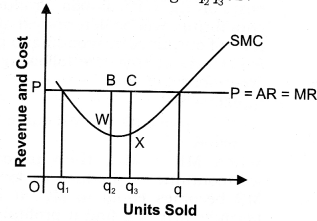(iii) Suppose now, that the firm reduce its output level from q2 to q1 The decrease in total revenue of the firm from this output is the market price multiplied by the change in quantity (ATR = market price x AQ), that is, the area of rectangle q2q3CB.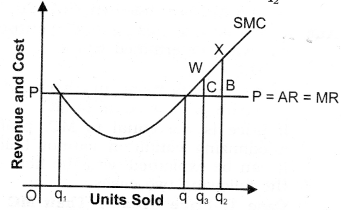(iv) On the other hand, the decrease in total cost with this decrease in output is the area of the region q2q3WX.
(v) But, a comparison of the two area shows, that by reducing the output from q2 to q3, the decrease in cost is more than the loss in revenue. So, q2 is not a profit maximizing level of output.

Question 3. Will a profit-maximizing firm in a competitive market ever produce a positive level of output in the range where the marginal cost is falling? Give an explanation.
[3-4 Marks]

Answer: No, as sufficient condition of producer equilibrium is Marginal Cost must be rising when Marginal Cost = Marginal revenue. It can be explained with the help of following diagram: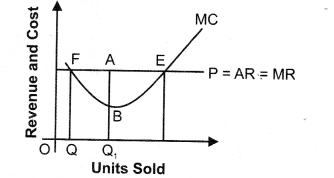Point F is not a producer equilibrium because at this point, marginal cost = marginal revenue when marginal cost is falling. It is so because after point F, and output then producer will continue to produce as long as MR becomes equal to MC as firm will find it profitable to raise the output level.

Question 4. The following table shows the total revenue and total cost schedules of a competitive firm. Calculate the profit at each output level. Determine also the market price of the goods. [3-4 Marks]

Question 5. The following table shows the total cost schedule of a competitive firm. It is given that the price of the goods is Rs. 10. Calculate the profit at each output level. Find the profit maximising level of output.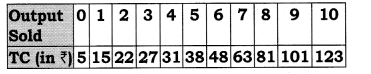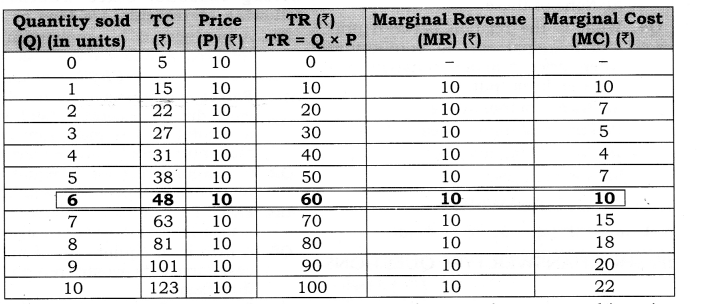The producer achieves equilibrium at 6 units of output because at this point MC = MR and MC is rising.

MORE QUESTIONS SOLVED

I. Very Short Answer Type Questions (1 Mark)
Question 1. What is meant by profit?
Answer: Profit refers to the excess of revenue over cost.

Question 2. What are the two methods for determination of producer’s equilibrium?
Answer: (i) TR – TC Approach
(ii) MR – MC Approach

Question 3. Explain the producer’s equilibrium. Or
Give meaning of producer’s equilibrium. [CBSE2004C, 05, 07C, 11, 11C;AI05, 08, 11}

Answer: A producer is said to be in equilibrium when he produces that level of output at which his profits are maximum.
Producer’s equilibrium is also known as profit maximisation situation.

Question 4. What is the general profit maximising condition for a producer (MR and MC approach)?
Answer:  (i) MC = MR; and
(ii) MC curve cuts the MR curve from below (i.e., MC is rising).

II. Multiple Choice Questions (1 Mark)
Question 1. If MC is more than MR at a particular level of output, how will the producer react to maximize the profits—:
(a) Decrease Production
(b) Increase Production
(c) Increase Revenue
(d) None of these

Question 2. When MC is equal to MR, while maximizing profit, then
(a) MC must be rising
(b) MC must be falling
(c) MC must be constant
(d) None of these

Question 3. What is the relation between price and marginal cost at equilibrium, when price falls with the rise in output.
(a) Price = Marginal Cost
(b) Price > Marginal Cost
(c) Price < Marginal cost
(d) None of these

Question 4. What is the relation between price and marginal cost at equilibrium, when price remains constant with the rise in output.
(a) Price = Marginal Cost
(b) Price > Marginal Cost
(c) Price < Marginal cost
(d) None of these

III. Short Answer Type Questions (3-4 Marks)
Question 1. What is the relation between Price and MC at equilibrium (when price falls with the rise in output)?

• When more output can be sold only by reducing the prices, AR or Price > MR.
• Equilibrium is achieved when MC = MR.
• So, Price is more than MC at the equilibrium level.

Question 2. “MC should be rising at the point of Producer’s Equilibrium”. Comment.

• The given statement is correct.
• If MC is falling at the point of equilibrium, it means that it is possible to add to profits by producing more,
• So, MC should be rising at the point of producer’s equilibrium.

Question 3. Find out the maximum profit position of a producer by comparing his MC and MR on the basis of the following data: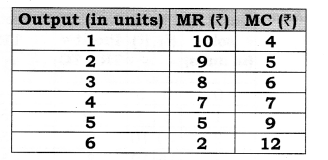Answer: The producer will be in equilibrium when MR = MC. It occurs at 4 units of output where both MR and MC are equal to 7.

IV. True Or False
Giving reasons, state whether the following statements are true, or false.
Question 1. A producer is in equilibrium when total cost and total revenue are equal.[CBSE, Foreign 2009]
Answer: False: A producer is in equilibrium when difference between total revenue and total cost is maximum so that maximum profit may be earned.

Question 2. If marginal revenue is equal to the total cost, producer is in equilibrium.
Answer: False: Producer is in equilibrium when marginal revenue is equal to marginal cost.

Question 3. A firm is in equilibrium if marginal cost curve cuts average revenue curve from below.
Answer: False: A firm is in equilibrium if marginal cost curve cuts marginal revenue curve from below.

Question 4. A firm gets maximum profits only if difference between average revenue and average cost is the maximum.
Answer: False: A producer is only in equilibrium if difference between total revenue and total cost is maximum.

Note: As per CBSE guidelines, no marks will be given if reason to the answer is not explained.

V. Long Answer Type Questions (6 Marks)
Question 1. Explain the producer’s equilibrium with MR/MC approach (when price falls with the rise in output).
Or
Explain producer’s equilibrium with the help of MC and MR schedules. Use diagram. [CBSE 2010,11 C; AI11]
Or
Why is the equality between marginal cost and marginal revenue necessary for a firm to be in equilibrium? Is it sufficient to ensure equilibrium? Explain. [CBSE 2015]

Answer: When there is no fixed price and price falls with the rise in output, MR curve slope downwards. Producer aims to produce that level of output at which MC is equal to MR and MC curve cuts the MR curve from below. Let us understand this with the help of following table:
According to Table, both the conditions of equilibrium are satisfied at 4 units of output.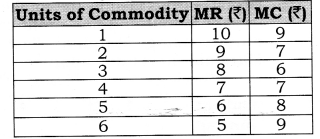MC is equal to MR and MC is rising. MC is more than MR when output is produced after 4 units of output. So, Producer’s Equilibrium will be achieved at 4 units of output. Let us understand the determination of equilibrium with the help of a diagram: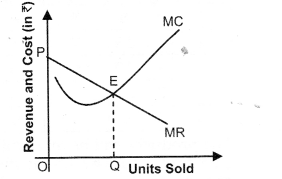Producer’s Equilibrium is determined at OQ level of output corresponding to point E as at this point, MC = MR and MC curve cuts MR curve from below. In Figure, output is shown on the horizontal axis and revenue and costs on the vertical axis. Producer’s equilibrium will be determined at OQ level of output corresponding to point E because at this, the following two conditions are met:
(i) MC = MR; and
(ii) MC curve cuts the MR curve from below.
When MR > MC, then producer will continue to produce as long as MR becomes equal to MC. It is so because firm will find it profitable to raise the output level.
When MR < MC, then producer will cut down the production as long as MR becomes equal to MC. It is so because firm will find it unprofitable to produce an extra unit. So, it starts reducing the level of output till MR = MC.
So, the producer is at equilibrium at OQ units of output.

Question 2. From the following schedule find out the level of output at which the producer is in equilibrium. Calculate profit. Give reasons for your answer. [CBSE Delhi 2009 (Set-1 &, III)]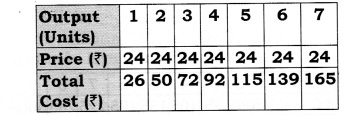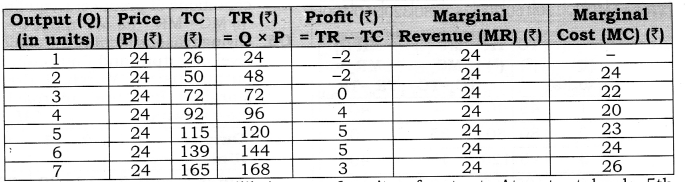The producer achieves equilibrium at 6 units of output. At output levels 5th and 6th unit, the difference between TR and TC, i.e., profit is maximum, which is equal to 5 in both the cases. But the producer is in equilibrium at the 6th unit only where MR = MC (= 24) and MC is rising.

Question 3. On the basis of the information given below, determine the level of output at which the producer will be in equilibrium. Use the marginal cost- marginal revenue approach. Give reasons for your answer.[CBSE Delhi, CBSE Sample Paper 2010]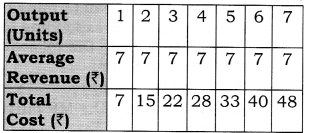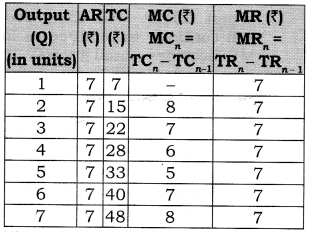The producer achieves equilibrium at 6 units of output. It is because this level of output satisfies both the conditions of producer’s equilibrium: (i) MC is equal to MR; and (ii) MC becomes greater than MR after this level of output.

Question 4. A table showing TC and TR of a firm is given. Calculate MC and MR and find out the equilibrium level of output.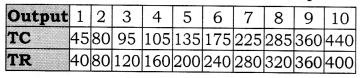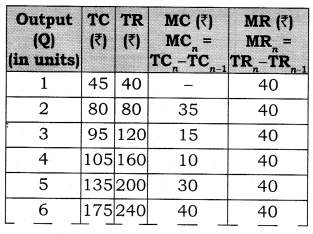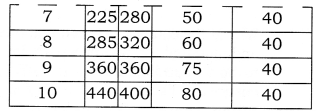The producer achieves equilibrium at 6 units of output. It is because this level of output satisfies both the conditions of producer’s equilibrium:
(i) MC is equal to MR.
(ii) MC becomes greater than MR after this level of output.

Question 5. Giving reasons identify the equilibrium level of output and find profit at this output using ‘Marginal Cost and Marginal Revenue’ approach from the following?